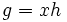# Tour:Left cosets are in bijection via left multiplication

PREVIOUS: Left cosets partition a group| UP: Introduction three (beginners)| NEXT: Right coset of a subgroup
General instructions for the tour | Pedagogical notes for the tour | Pedagogical notes for this part
The different left cosets of a subgroup are in bijection with each other, under the action of the group via left multiplication. This means that all left cosets of a subgroup are of the same size.
PREREQUISITES: Definition of group, subgroup and coset, invertible implies cancellative, and Tour:Mind's eye test two (beginners)#Left and right multiplication maps
WHAT YOU NEED TO DO: Read and understand the statement and proof below. If you find it hard, refer back to the suggested prerequisites pages.

## Statement

### Statement with symbols

Let$H$ be a subgroup of a group$G$ and let$xH$ and$yH$ be two left cosets of$H$. Then, there is a bijection between$xH$ and$yH$ as subsets of$G$, given by the left multiplication by$yx^{-1}$.

## Facts used

1. Invertible implies cancellative in monoid: In particular, we can cancel elements in a group: if$ab = ac$, then$b = c$.

## Proof

Given: A group$G$, a subgroup$H$, and two left cosets$xH$,$yH$ of$H$

To prove: Left multiplication by$yx^{-1}$ establishes a bijection between$xH$ and$yH$.

Proof: We prove that left multiplication by$yx^{-1}$ sends$xH$ to$yH$, is surjective, and is injective.

1. Well-defined as a map from$xH$ to$yH$: First, note that if$g = xh$ then$yx^{-1}g = yh$. Thus, any element in$xH$ gets mapped to an element in$yH$.
2. Surjective: Every element of the form$yh$ with$h$ in$H$ arises as$yx^{-1}(xh)$, hence, it arises as the image of left multiplication by$yx^{-1}$. Thus, the map from$xH$ to$yH$ is surjective.
3. Injective: Given two distinct elements$xh_1, xh_2 \in xH$, the elements$(yx^{-1})xh_1 = yh_1$ and$(yx^{-1})xh_2 = yh_2$ are also distinct, because by fact (1), if they were equal, then canceling$yx^{-1}$ from both sides would give$xh_1 = xh_2$. Thus, left multiplication by$yx^{-1}$ sends distinct elements to distinct elements, so the map is injective.

Thus, left multiplication by$yx^{-1}$ is a bijection from$xH$ to$yH$.

### Sidenote

In general, there is no natural bijection between two left cosets -- the bijection depends on a choice of element in both cosets (the elements$x$ and$y$ in the above description).